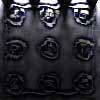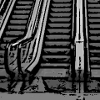#### You may also like### Fixing the Odds

You have two bags, four red balls and four white balls. You must put all the balls in the bags although you are allowed to have one bag empty. How should you distribute the balls between the two bags so as to make the probability of choosing a red ball as small as possible and what will the probability be in that case?### Scratch Cards

To win on a scratch card you have to uncover three numbers that add up to more than fifteen. What is the probability of winning a prize?### Escalator

At Holborn underground station there is a very long escalator. Two people are in a hurry and so climb the escalator as it is moving upwards, thus adding their speed to that of the moving steps. ... How many steps are there on the escalator?

# In Constantly Passing

##### Age 14 to 16Challenge Level

Thank Justin from Skyview High School, Billings, MT, USA for this solution and well done!

Rate, time and distance are connected by the equation r =d/t .

Call the rate (or speed) of the car r c and the rate of every bus r b . Each bus is a constant distance from the bus preceding it and the bus following it; call this distance d.

For a bus approaching on the other highway and coming towards the car, the rate of the bus relative to the car, considering the bus still, is (r b + r c ). This rate multiplied by the time it takes (three minutes) for the car to close the gap between it and the bus is equal to d, hence:

3(r b + r c ) = d.

The rate of the bus which is travelling in the same direction as the car, relative to the rate of the car (considering the car still) is (r b - r c ). This rate multiplied by the time it takes (six minutes) for the bus to close the gap between it and the car is also equal to d 1 , hence

6(r b - r c ) = d.

Multiplying the first equation by 2 and add the two equations, one obtains

12r b = 3d.

But the distance d between the buses divided by the rate of the bus is equal to the time interval between the buses therefore the time interval = d/r b = 4 minutes.

So the buses leave the depot at intervals of 4 minutes.